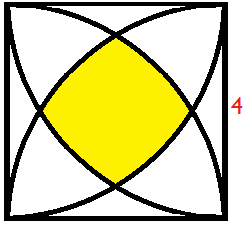# Slightly inflated square

Geometry Level 4The figure above consists of 4 identical intersecting quadrants inside a square of side 4. The area of the shaded region can be expressed as $\frac{a}{b}\pi+c-d\sqrt{e}$ for positive integers $a,b,c,d$ with $e$ with $a$ and $b$ are relatively coprime, and $e$ is square free.

Evaluate the value of $a+b+c+d+e$.

×